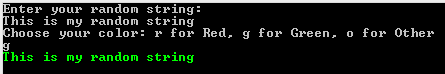In this article, we are going to talk about conditions in C#. We will learn how to write simple conditional statements, nested conditional statements, and multiple conditional statements.

If you want to download the source code for our examples, you can do that from here Conditions in C# Source Code.

For the complete navigation of this series check out: C# Back to Basics.

Let’s begin.

## Basic Conditions in C#

If we want to execute some expression but only if some condition is met, then we need to use conditional statements. To create such a statement we need to use `if` and `else` keywords:

```if (condition)
{
< expression1 > ;
}
else
{
< expression2 > ;
}
```

The condition is a logical expression that can result in true or false. If it is true then the `<expression1>` will be executed, otherwise, `<expression2>` will be executed. After every expression, we need to place the `;` sign.

We can execute more expressions if the condition is true or false:

```if (condition)
{
< expression1 > ;
< expression2 > ;
}
else
{
< expression3 > ;
< expression4 > ;
}
```

Example 1: Create an application that determines the greater number of two integer inputs:

```class Program
{
static void Main(string[] args)
{
Console.WriteLine("Enter the first number: ");

Console.WriteLine("Enter the second number: ");

if(first > second)
{
Console.WriteLine(\$"The greater number is {first}");
}
else
{
Console.WriteLine(\$"The greater number is {second}");
}

}
}
```

The result:

```Enter the first number:
92
Enter the second number:
36
The greater number is 92```

We don’t have to use only `if` and `else` keywords in conditional statements, we can add another condition by adding `else if` block part:

```if(condition1)
{
< expression 1 > ;
}
else if(condition 2)
{
< expression 2 > ;
}
else if(condition n)
{
<expression n>
}
else
{
< expression k > ;
}
```

Example 2: Create an application that takes any string and the font color (r for red, g for green, o for other) as inputs. Then it needs to print out that string with the selected color:

```class Program
{
static void Main(string[] args)
{

Console.WriteLine("Choose your color: r for Red, g for Green, o for Other");

if(color == 'r')
{
Console.ForegroundColor = ConsoleColor.Red;
Console.WriteLine(sentence);
}
else if(color == 'g')
{
Console.ForegroundColor = ConsoleColor.Green;
Console.WriteLine(sentence);
}
else
{
Console.ForegroundColor = ConsoleColor.Blue;
Console.WriteLine(sentence);
}

}
}
```## Nested Conditions in C#

In C#, we can write a conditional statement inside a conditional statement if that’s one of the requirements of our project. So, the base syntax looks like this:

```if (condition)
{
if (condition2)
{
< expression1 > ;
}
else
{
< expression2 > ;
}
}
else
{
< expression3 > ;
}
```

Even though we can create nested conditional statements, we do not recommend them that much, because it would lead to low readability.

Example 3: Create an application in which the user enters a number between 1 and 100. If the number is lower than 50, our application will output multiplication by 5. But if a number is greater than 50 then for even number application will output multiplication by 2 and for an odd number application will output multiplication by 3:

```class Program
{
static void Main(string[] args)
{

if (number > 50)
{
if(number % 2 == 0) //reminder in division with two for even numbers is always a zero.
{
Console.WriteLine(number * 2);
}
else
{
Console.WriteLine(number * 3);
}
}
else
{
Console.WriteLine(number * 5);
}

}
}
```

The result:

```Enter your number:
68
136```

## Switch-Case Statements

In a situation where we need more than one or two conditions to execute some expression, using multiple branching could be an advantage. To use multiple branching in C#, we need to use `switch` and `case` keywords:

```switch (expression)
{
case value1:
<expression 1> ;
break;
case value2:
<expression 2> ;
break;
default:
< expression3>;
break;
}
```

Example 4: Create an application that accepts month number as an input and prints out the number of days in that month:

```static void Main(string[] args)
{
Console.WriteLine("Enter the month number from 1 to 12");

switch (month)
{
case 1: case 3: case 5:
case 7: case 8:
case 10: case 12:
Console.WriteLine("Number of days is 31");
break;
case 4: case 6:
case 9: case 11:
Console.WriteLine("Number of days is 30");
break;
case 2:
Console.WriteLine("Number of days is 28 or 29");
break;
default:
Console.WriteLine("Your number is not between 1 and 12");
break;
}

}
```

The result:

```Enter the month number form 1 to 12
9
Number of days is 30```

## Conclusion

We this knowledge, we can create conditional structures in our code and make decisions based on whether the conditions are true or false.

In our next post of this series, we are going to talk about Loops in C#.